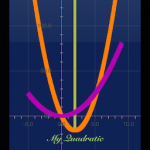For a long time, I have nurtured a dream of creating an interactive graphing Math book. Mathematics is a subject that provides solutions to life problems from simple purchases at the local market to the complex flight paths of spaceships to other planets in the universe; from the concentration computations of chemical solutions in the laboratory to the compound interest calculations at the financial institution.

Mathematical theory is rather difficult to understand if there is no way to visualize how it looks and feels. Models and graphs help. Interactive models and graphs will definitely bring enjoyment to studying.

With the continuous advancements in mobile computing, an interactive graphing Math book can now be realized where mathematical theory with interactive graphing features can be in the hands of hundreds of millions of people in the world, anywhere any time. My dream has come true with the first iPhone app – “My Quadratic”, and more educational and scientific apps to come. My Quadratic app can be downloaded from this link:

The world is really flat. Education must be universal!

Thinh M. Le, Ph.D.

April 8, 2016

• Version 3.5: Supports iOS 9.3 and above. Addition of practice questions.
• Version 3.4: iOS 10.3. iPhone 7plus, 7, SE, 6s plus, 6s, 6 plus, 6, 5s, 5. iPad Air, Pro, Retina.
• Version 3.3: iOS 10.0. iPhone 7plus, 7, SE, 6s plus, 6s, 6 plus, 6, 5s, 5. iPad Air, Pro, Retina.
• Version 3.2: Supports for iPad.
• Version 3.1: Examples are added to the section on finding zeros using Sum and Product. Minor layout fix..
• Version 3.0: Addition of Finding Zeros using Product and Sum, Addition of practice questions.
• Version 2.0: Support of iOS 9.2, Section on Converting from Factored Form to Vertex and General Forms added.
• Version 1.0: This app is an interactive graphing math book in which high school, college students and science lovers can observe and try out various ways of analyzing a quadratic function with graphing features. Students are gradually introduced the theory behind a quadratic function with various parameters. Mathematically, there are three forms in a quadratic function – a polynomial of order 2: the vertex form, the general form, and the factored form. This app shows techniques to find the zeros, the coordinates of the vertex, the y-intercept; to draw the corresponding parabola and its axis of symmetry; as well as to algebraically convert from one form to another. There are formula-specific calculators (CalPad) and parabola plotter (PlotPad) that help students characterize a quadratic function with domain, range, and the variations; and visualizing the relative size and shape of the transformed parabola compared to the basic one.
• The vertex form: f(x) = a(x – h)² + k
• The general form: f(x) = ax² + bx + c
• The factored form: f(x) = a(x – x₁)(x – x₂).